# Methods and formulas for Mann-Whitney Test

Select the method or formula of your choice.

## Test statistic

Minitab calculates the Mann-Whitney statistic as follows:
1. Minitab ranks the two combined samples. Minitab gives the smallest observation rank 1, the second smallest observation rank 2, and so on.
2. If two or more observations are tied, Minitab assigns the average rank to both observations.
3. Minitab sums the ranks of the first sample.

## Ranking tied values

Tied values occur when two or more observations are equal. If your data has tied values, Minitab ranks the data as follows:
1. Sort the observations in ascending order.
2. Assign ranks to each observation as if there were no ties.
3. For a tied set, take the average of the corresponding ranks and assign this value as the new rank to each tied value in that set.

### Example

A sample has 9 observations: 2.4, 5.3, 2.4, 4.0, 1.2, 3.6, 4.0, 4.3, and 4.0.

Observation Rank

(assuming no ties)

Rank
1.2 1 1
Tied 2.4 2 2.5
2.4 3 2.5
3.6 4 4
Tied 4.0 5 6
4.0 6 6
4.0 7 6
4.3 8 8
5.3 9 9

Minitab also uses the following information to calculate the test statistics:
• The number of the set of ties is 2.
• The number of tied values that is in the 1st set is 2.
• The number of tied values that is in the 2nd set is 3.

## P-value

### Formula

The Mann-Whitney test uses a normal approximation method to determine the p-value of the test.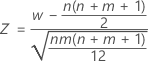is approximately distributed as a normal distribution with a mean of 0 and a standard deviation of 1, N(0,1).

The normal approximation p-value for the three alternative hypotheses uses a continuity correction of 0.5.
Alternative hypothesis P-value
H1: η1 > η2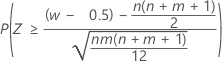H1: η1 < η2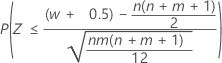H1: η1 ≠ η2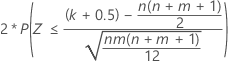If your data contains ties, then, Minitab adjusts the p-value by replacing the denominator of the above Z statistics by:

The adjusted p-value is usually more accurate than the unadjusted p-value. However, the unadjusted p-value is the more conservative estimate because it is always greater than the adjusted p-value for a specific pair of samples.

### Notation

TermDescription
WMann-Whitney test statistics
nsize of sample 1
msize of sample 2
η1median of sample 1
η2median of sample 2
k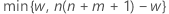i1, 2, …, I
Inumber of sets of ties
tinumber of tied values in the ith set of ties

## Point estimate

The approximation algorithm that Minitab uses to calculate the point estimate of η1η2is described in this article: J.W. McKean and T.A. Ryan, Jr. (1977). "An Algorithm for Obtaining Confidence Intervals and Point Estimates Based on Ranks in the Two Sample Location Problem", Transactions on Mathematical Software, 183–185.

## Confidence interval

The confidence interval of η1 – η2 is defined as the range of values of η1 – η2 for which the null hypothesis is not rejected.

The method that Minitab uses to calculate the confidence interval is described in this article: J.W. McKean and T.A. Ryan, Jr. (1977). "An Algorithm for Obtaining Confidence Intervals and Point Estimates Based on Ranks in the Two Sample Location Problem", Transactions on Mathematical Software, pp.183-185.

By using this site you agree to the use of cookies for analytics and personalized content.  Read our policy